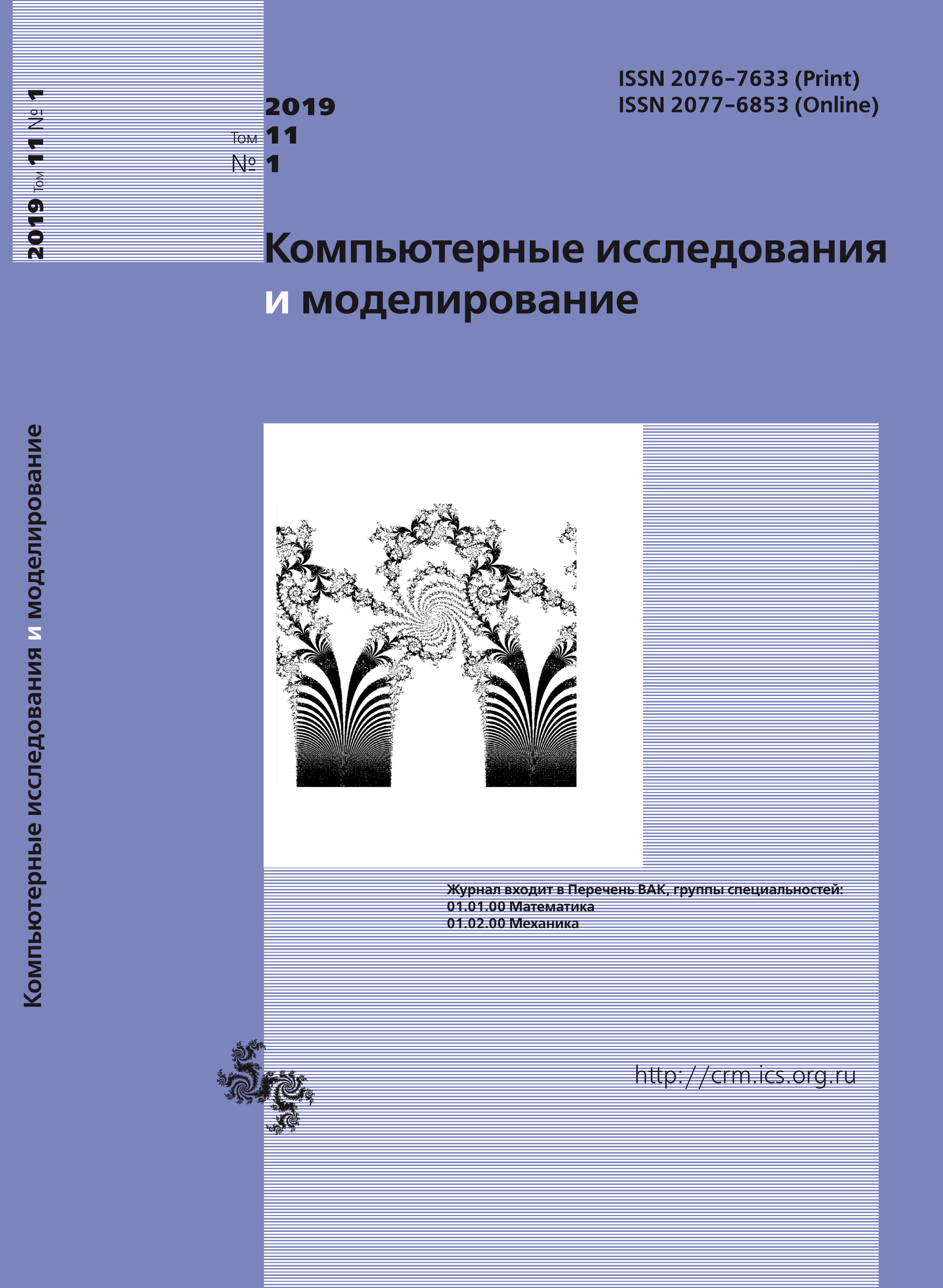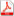Issue 1, 2019 Vol. 11

# All issues

Weighthed vector finite element method and its applicationspdf (2112K)  / List of references

Mathematical models of many natural processes are described by partial differential equations with singular solutions. Classical numerical methods for determination of approximate solution to such problems are inefficient. In the present paper a boundary value problem for vector wave equation in L-shaped domain is considered. The presence of reentrant corner of size $3\pi/2$ on the boundary of computational domain leads to the strong singularity of the solution, i.e. it does not belong to the Sobolev space $H^1$ so classical and special numerical methods have a convergence rate less than $O(h)$. Therefore in the present paper a special weighted set of vector-functions is introduced. In this set the solution of considered boundary value problem is defined as $R_ν$-generalized one.

For numerical determination of the $R_ν$-generalized solution a weighted vector finite element method is constructed. The basic difference of this method is that the basis functions contain as a factor a special weight function in a degree depending on the properties of the solution of initial problem. This allows to significantly raise a convergence speed of approximate solution to the exact one when the mesh is refined. Moreover, introduced basis functions are solenoidal, therefore the solenoidal condition for the solution is taken into account precisely, so the spurious numerical solutions are prevented.

Results of numerical experiments are presented for series of different type model problems: some of them have a solution containing only singular component and some of them have a solution containing a singular and regular components. Results of numerical experiment showed that when a finite element mesh is refined a convergence rate of the constructed weighted vector finite element method is $O(h)$, that is more than one and a half times better in comparison with special methods developed for described problem, namely singular complement method and regularization method. Another features of constructed method are algorithmic simplicity and naturalness of the solution determination that is beneficial for numerical computations.

Keywords: weighted vector FEM, weighted spaces, $R_ν$-generalized solution, boundary value problems with singularity
Citation in English: Rukavishnikov V.A., Mosolapov A.O. Weighthed vector finite element method and its applications // Computer Research and Modeling, 2019, vol. 11, no. 1, pp. 71-86
Citation in English: Rukavishnikov V.A., Mosolapov A.O. Weighthed vector finite element method and its applications // Computer Research and Modeling, 2019, vol. 11, no. 1, pp. 71-86
DOI: 10.20537/2076-7633-2019-11-1-71-86
Views (last year): 37.

Full-text version of the journal is also available on the web site of the scientific electronic library eLIBRARY.RU

The journal is included in the Russian Science Citation Index

The journal is included in the List of Russian peer-reviewed journals publishing the main research results of PhD and doctoral dissertations.

International Interdisciplinary Conference "Mathematics. Computing. Education"

The journal is included in the RSCI

Indexed in Scopus